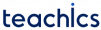# Bernoulli’s Principle and The Continuity Equation

Flow meters are the devices used for measuring the flow rate of liquids or gases. They work on Bernoulli’s principle and continuity equation.

## The Continuity equation

The continuity equation represents that the product of the cross-sectional area of the pipe and the velocity of the fluid at any point is always a constant.

Consider the fluid flows from end A, having a cross-sectional area A1, to end B with a cross-sectional area A2 in the pipe. Then we can write,In general form, we can say## Bernoulli’s Principle

Bernoulli’s principle states that,

The total mechanical energy of the moving fluid comprising the gravitational potential energy of elevation, the energy associated with the fluid pressure and the kinetic energy of the fluid motion, remains constant.

The formula for Bernoulli’s principle is given byWhere,
P – Pressure exerted by the fluid.
v – Velocity of the fluid.
ρ – Density of the fluid.
h – Height of the container.

Subscribe
Notify of